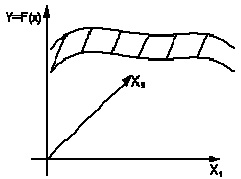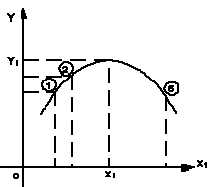Mixture formation plays
9

How to evaluate the accuracy of representation response function?

• How to use the presentation to find the optimal values of Y?

Geometric representation response function in the factor space X1, X2, ..., n is called surface response (Fig.1.1.1).Figure 1.1.1: Surface review

If Influence on Y only one factor X1, the response function of the – pretty simple Y and graph Y = F (X) (Figure 1.1.2). With it you can customize the look of the mathematical expression response function. If we do not believe that the experiments are reproduced well, it is usually repeated several times experiments and dependence are given spread empirical data:Figure 1.1.2: Construction of the response function of one variable on experience in this

If two factors, it is necessary to conduct experiments at different ratios of these factors. The resulting response function in 3–dimensional space (Fig. 1.1.1) can be analyzed by conducting a series of cross sections at fixed values of other